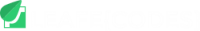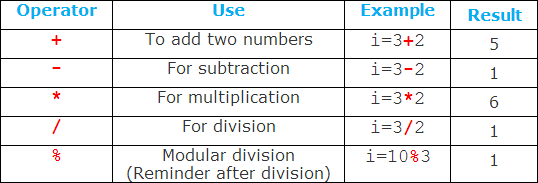# B4A for beginners – Math Operators

## B4A Tutorials on Math Operators

Math Operators are used to perform operations, for example if you define a variable like this:

Dim purchases As Double = 245.00
Dim discount As Double = 15.00
Dim totalAmount As Double

purchases = 245.00
discount = 15.00

Now before you can get  the total amount payable, you have to subtract the discount from the purchases as in the example below. You will find out that we used Subtraction to perform the operation.

totalAmount = purchases – discount

Other Maths Operators are: